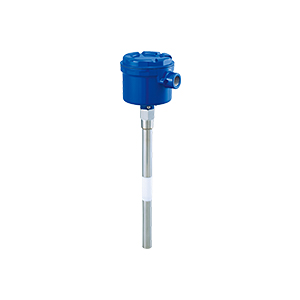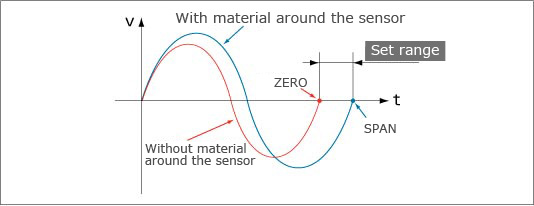1 Butuh Bantuan?

## Main application

Virtually any liquids, solids and bulk solids## Principle of Operation

The basic oscillator circuit is of the parallel resonance circuit with L (coil) and C (capacitance between the electrodes).
The oscillation frequency (f) of this circuit is : f = 1/2 π √LC.
The frequency without material around the sensor (f1) is: f1 = 1/2 π √LC, where C is the capacitance without material around the sensor (zero point).
With material around the sensor, the capacitance increases (C+ΔC), and the frequency (f2) is: f2 = 1/2 π √L(C+ΔC), where C+ΔC is the capacitance with material around the sensor (span point).
The sensor detects the frequency change from f1 to f2, and actuates the relay. The frequency range is divided into 8 segments (between zero and span), and the set and reset points assigned on a desired segment, which means high limit, low limit and hysteresis can be programmed.Set range is divided into 8 segments, and ON/OFF points are set for the segments. Hysteresis is also programmable.